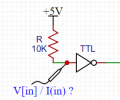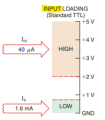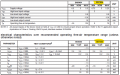# Pull up resistor - how Voltage & Current are determined ?

#### 2Hexornot2Hex

Joined Apr 16, 2020
29
Hi Gurus,

Pull up resistor is a well known concept.
Probably, after reading on this too much, I totally lost it and confused - how it works on a basic levelWhen we place a certain pull up resistor (R), how the actual Voltage and Current are being determined ?
The well known formula $$U=I*R$$ has 2 unknown variables (U & I).

Assume we have the following setupThe TTL IC has the following INPUT definitions:While calculating, I can pick different values of V[in] and find the relevant I[in]....but how actually the setup will behave ?
(Vcc=5V; R=10K)
Case NoV[in]
(picked by me for the following calculations)
Vr
(voltage dropped by R= Vcc-Vin)
I[in]
(=Vr / R)
14.6V0.4V40uA
24.0V1V100uA
30.2V4.8V480uA
For convenience, from SN74LS04 datasheet:#### BobTPH

Joined Jun 5, 2013
2,388
The datasheet tells you the current drawn by an input pin at 5.5V (1ma) and at 2.4V (40uA). From this you should be able to calculate the max resistor you can use to guaratee the input is at 2.4V, which is safely above the minimum 2.0V fir a high input.

Bob

#### panic mode

Joined Oct 10, 2011
1,829
TTL inputs will float high if left not connected which is obvious from internal schematics that can be found in datasheets. but this means input impedance is relatively high and therefore can be noise sensitive. adding pullup resistor can reduce that impedance and "stiffen" the input.

about listed values - observe sign (current direction). negative means current is leaving pin and flowing through external circuit to GND. and this is the reason not connected TTL input will float high.

you need to have an idea about impedance of that external circuit pulling the pin low. then you can size pullup resistor that will keep pin above threshold.

#### 2Hexornot2Hex

Joined Apr 16, 2020
29
TTL inputs will float high if left not connected....
...
about listed values - observe sign (current direction). negative means current is leaving pin and flowing through external circuit to GND. and this is the reason not connected TTL input will float high.
...
you need to have an idea about impedance of that external circuit...
You answered some questions but certainly not mineThe question is not regarding whether pull up resistors are required or what would happen if it wasn't connected ...
I provided a specific circuit.

#### Janis59

Joined Aug 21, 2017
1,178
Need to know static current consumption at logic 1 and inner parasite capacitance. So, outer pullup resistor with equivalent inner resistor made by current consumption (even if very small) makes a resistive voltage divider where one must fall-into the datasheet logical 1 voltage boarders. At another hand, while a dynamic regime, say pulse, the inner capacitance with a pullup resistor plus small inductance of pcb patches and resistor parasite inductance itself forms the delay line, so there must be set the goal how long delay is acceptable. Sure, for finger pushed button it may be hundreds of msec in worst case, but when it is 16 MHz driven i2c or anything else, that must be hard under microsecond.

#### MrChips

Joined Oct 2, 2009
21,076
Suppose Vcc = 5V
Suppose that you want to guarantee Vin = 4V
Suppose IIH = 40μA

Rpullup = (5V - 4V) / 40 μA = 1/40 MΩ = 25kΩ

#### 2Hexornot2Hex

Joined Apr 16, 2020
29
The datasheet tells you the current drawn by an input pin at 5.5V (1ma) and at 2.4V (40uA). From this you should be able to calculate the max resistor you can use to guaratee the input is at 2.4V, which is safely above the minimum 2.0V fir a high input.
I'm familiar with pull up R value calculation technique.
I'm trying to figure out how this circuit behaves with a given R. How it's being "decided" what Voltage and Current levels would be consumed by the TTL IC in my circuit.

#### MrChips

Joined Oct 2, 2009
21,076
Look into the device datasheet and find the value for IIH.

#### WBahn

Joined Mar 31, 2012
25,745
I'm familiar with pull up R value calculation technique.
I'm trying to figure out how this circuit behaves with a given R. How it's being "decided" what Voltage and Current levels would be consumed by the TTL IC in my circuit.
It's being "decided" by the intersection of the load lines for the pullup resistor and the input circuitry of the chip.

Imagine taking your pullup resistor and connecting it to an adjustable voltage source instead of the chip input. If the output of this supply was equal to the power rail, no current would flow in the resistor. As you lower the voltage, current flows. The more you lower the voltage, the more current that flows. This relationship is pretty well known because resistors are pretty well behaved. Now do the same for the chip input. In general, as you raise the voltage the more current that you will have flowing. This relationship, however, is not very well known, especially for a specific chip, because it depends on a lot of different variables and is generally quite nonlinear. But it is what it is and when you connect the resistor to the chip the voltage that will result at the chip input is the voltage that corresponds to the same current flowing in the resistor that is flowing in the chip.

What the data sheet tells us is not what that relationship is for the chip, but what some of the boundaries are. It's saying that at at the critical voltages the resulting current will be no more than a certain amount (under certain conditions). We can then use this to size resistors so that we have assurance that our voltage levels will always be such as to establish valid logic levels at the inputs.

#### 2Hexornot2Hex

Joined Apr 16, 2020
29
Look into the device datasheet and find the value for IIH.
I see it clear that I[IH] is maximum 40uA at V[in] of 2.4 V, but how does it help understand what actual Vin and I will be with the provided R ?
I described 3 hypothetical 'on-paper' cases (where different pairs of Vin and I could occur, by Ohm's law) - but what decides about actual V and I values ?
Confused...

#### andrewmm

Joined Feb 25, 2011
314
The input circuit of a logic is none linear,
For most logic design, this none linearity is not relevant to design.

The input circuits of digital chips are amazingly complicated, current sources, esd protection, current mirrors , equivalent resistance et all. The pictures you see in data sheets are very very simplified ,

Just to add confusion, traditional TTL chips source current out of there inputs, CMOS chips don't ( mainly )

but if you want to know more about input pin characteristics, then the answer is IBIS models,
these can be used in circuit simulators to give you the answer you desire.

#### 2Hexornot2Hex

Joined Apr 16, 2020
29
... and when you connect the resistor to the chip the voltage that will result at the chip input is the voltage that corresponds to the same current flowing in the resistor that is flowing in the chip.
The Voltage is being determined by Current, And how it's being "decided" what current should flow ?

#### MrChips

Joined Oct 2, 2009
21,076
I see it clear that I[IH] is maximum 40uA at V[in] of 2.4 V, but how does it help understand what actual Vin and I will be with the provided R ?
I described 3 hypothetical 'on-paper' cases (where different pairs of Vin and I could occur, by Ohm's law) - but what decides about actual V and I values ?
Confused...
Datasheets do not provide actual values because that can vary from device to device.
They will show statistically measured values within a range, such as minimum, maximum and typical.
Be careful how you interpret minimum and maximum values. These are manufacturer's guarantee that their device will perform if these limits are not exceeded. Depending on the parameter in question, you may find typical values are outside the minimum and maximum values. Confusing? Yes. You have to know how to interpret the data.

With the device in question, the maximum input current is 40μA. This does not say that the current will be 40μA.
As a design engineer you learn how to use this data. The datasheet states that the test condition was VI = 2.4V.
In the first graphic you showed that a valid logic-HIGH range is 2V-5V with IIH = 40μA.
VI = 2.4V does not give you much room for error. That is why I chose a value of VI = 4V as an example.

Rpullup = (5V - 4V) / 40 μA = 1/40 MΩ = 25kΩ

This calculation says that if I choose a pullup resistor = 25kΩ the input voltage will be 4V or higher so long as the input current does not exceed 40μA.

If I choose VI = 4.6V
Rpullup = (5V - 4.6V) / 40 μA = 0.4/40 MΩ = 10kΩ

Thus 10kΩ pullup gives even higher VI value so long as IIH does not exceed 40μA.

To summarize, the datasheet does not provide you with exact current and voltage. It allows you to design your circuit so that your circuit has good noise immunity while still operating within the specifications of the device.

#### WBahn

Joined Mar 31, 2012
25,745
The Voltage is being determined by Current, And how it's being "decided" what current should flow ?
The same way that it is decided how much current should flow in two resistors that are in series.

Current and voltage have a relationship that is imposed by the physics of the devices involved.

#### BobaMosfet

Joined Jul 1, 2009
1,108
@2Hexornot2Hex
You ask a good question. Here is the simple truth: A pull up or pull down resistor's sole purpose is to pull the voltage to a specific level WITHOUT impacting the circuit too much, and only in the absence of a primary signal on the conductor the pull-x is in parallel with.

In other words, it's about current. In order for voltage to be dropped, you have to make current flow. A weak pull-x resistor has a high value- that means it takes a higher amount of voltage dropped across it to make a very small amount of current flow. It's called weak, because it only causes a little current to flow across it. If your pull-x resistor allows too much current to flow, it will over-ride whatever other 'signal' level is on the conductor you're trying to influence- you don't want that. Remember, a pull-x resistor's job is to _influence_ another conductor, not control it.

Simple example:

MCU pin outputs a 1mA @ 5VDC signal. By definition, therefore, the pull-x resistor must allow _less_ current across itself than the MCU pin is outputting, otherwise, it won't matter what the MCU pin does, the pull-x resistor would override it.

so, in this case, we know that a 4.7K-Ohm resistor will give us about 1mA across it at 5VDC (0.00106A = 5/4700). So we want something higher in value (aka weaker in current pull): Let's try 100K-Ohm.

5/100000 = 0.00005A or 50uA. That's very small, but still enough to influence the MCU pin when the MCU pin is not placing a signal on that pin.

#### OBW0549

Joined Mar 2, 2015
3,393
I see it clear that I[IH] is maximum 40uA at V[in] of 2.4 V, but how does it help understand what actual Vin and I will be with the provided R ?
The reason you're not getting your question answered is because none of us who've actually designed with digital logic have ever had to concern ourselves with the issue; standardized digital logic families are intended to relieve us of the need to calculate exact voltages, currents and resistances for pullup resistors.

TTL inputs source current and will naturally pull themselves up to a logic HIGH level. But left open-circuit they may not do so reliably under all conditions, especially when a lot of noise is present in the vicinity. So we assist them with a pullup resistor, just to make sure. The value of resistance is not critical-- anything between 1 kΩ and 10 kΩ is almost certain to suffice-- and we don't waste our time, and our employer's money, fussing over the exact voltages and currents involved. Because it simply doesn't matter.

•cmartinez

#### 2Hexornot2Hex

Joined Apr 16, 2020
29
Depending on the parameter in question, you may find typical values are outside the minimum and maximum values. Confusing? Yes. You have to know how to interpret the data
You nailed it regarding confusion on this....I saw in several datasheets the Typical value falls outside the declared min/max. At first I thought it a typo, but since it occurred in several places I wanted to post a question on this later.
So the datasheet's '40 μA' is being used in all related pull-up calculations just because other Current level values (of this chip) are unknown to us, am I correct ?

#### 2Hexornot2Hex

Joined Apr 16, 2020
29
In other words, it's about current. In order for voltage to be dropped, you have to make current flow.
I think generally it's vise versa....the voltage difference causes current to flow

Simple example:

MCU pin outputs a 1mA @ 5VDC signal. By definition, therefore, the pull-x resistor must allow _less_ current across itself than the MCU pin is outputting, otherwise, it won't matter what the MCU pin does, the pull-x resistor would override it.

so, in this case, we know that a 4.7K-Ohm resistor will give us about 1mA across it at 5VDC (0.00106A = 5/4700). So we want something higher in value (aka weaker in current pull): Let's try 100K-Ohm.

5/100000 = 0.00005A or 50uA. That's very small, but still enough to influence the MCU pin when the MCU pin is not placing a signal on that pin.
The 'simple example' is unclear. In your calculations you're dropping all 5V across the resistor....and MCU pin is used as input & output.
In the original post I attached a very simple schematics (simpler than MCU's), you can reference it.

#### 2Hexornot2Hex

Joined Apr 16, 2020
29
I understand that it's 'a mystery' how the circuit will actually behave. The levels of actual V[in] & I[in] are unknown and can't be calculated (even from the info provided in datasheet).
We kind of 'rely' that the input will be pulled-up/down to HIGH/LOW (but to some actually 'unknown' level), based on calculation techniques for R values that worked previously fine in other designs.
Am I right ?#### MrChips

Joined Oct 2, 2009
21,076
It is not a "mystery".
Install a 10kΩ pullup resistor into your circuit. Then take a DMM and measure the voltage across the resistor.
This will allow you to determine both input current and voltage. Compare your results with the design specifications.

•atferrari and OBW0549